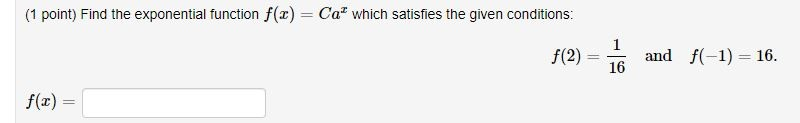## When Helping Means Winning contest

Ask a school-related question in the Help Zone and you could win a \$1000 scholarship!

# Help Zone

### Student Question

I am unable to do this problem. I keep getting weird numbers.Mathematics## Explanations (1)

•Explanation from AlloprofExplanation from Alloprof

This Explanation was submitted by a member of the Alloprof team.

Hello,

The exponential function is given by $$f(x)=Ca^x$$

You want to find the values for C and a.

You have f(2)=1/16. This means that when x=2, f(x)=1/16. Also, f(-1)=16 means that when x=-1, f(x)=16.

You can plug in the numbers in the general formula. You will now have two equations with two unknown variables.

\begin{align} f(2) &= Ca^2 \\ \frac{1 }{16 } &= Ca^2\\ \end{align}

\begin{align} f(-1) &= Ca^{-1 } \\ 16 &= Ca^{-1 }\\ 16 &= \frac{C }{a} \\ 16a &= C \\ a &= \frac{C }{16} \\ \end{align}

You isolated a and can plug that into the first equation that you found for f(2) to find a value for C.

\begin{align} \frac{1 }{16 } &= Ca^2\\ \frac{1 }{16 } &= C ( \frac{C }{16 } ) ^2 \\ … &= C \\ \end{align}

One you find the value for C, you can find the value for a.

An easy way would be to go back to a = C/16.

After finding the values for C and a, you will be able to give the exponential function $$f(x)=Ca^x$$ that satisfies the conditions.

Let us know if you have any more questions!